# Pandas中你一定要掌握的時間序列相關高級功能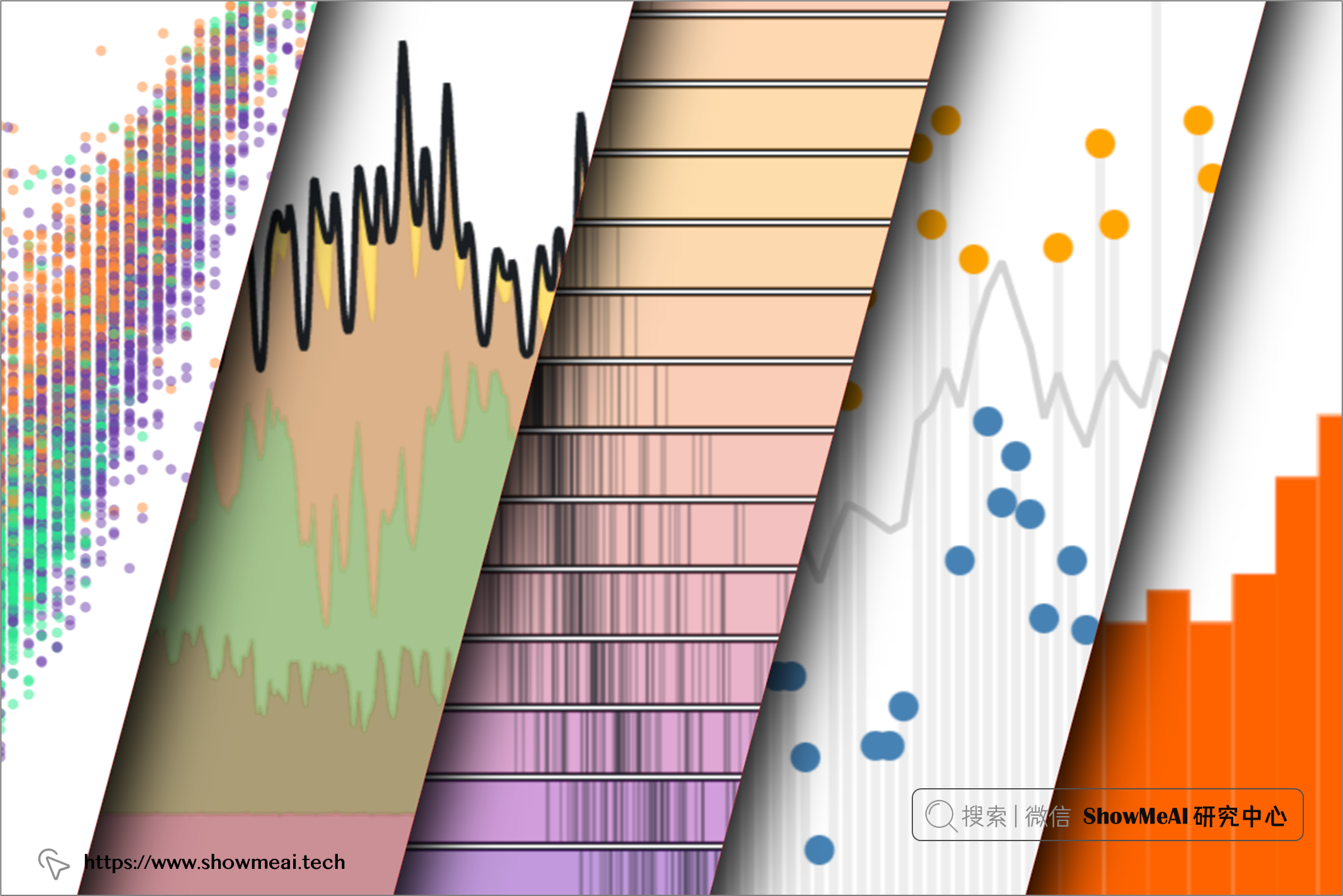Pandas 是大家都非常熟悉的數據分析與處理工具庫，對於結構化的業務數據，它能很方便地進行各種數據分析和數據操作。但我們的數據中，經常會存在對應時間的字段，很多業務數據也是時間序組織，很多時候我們不可避免地需要和時間序列數據打交道。其實 Pandas 中有非常好的時間序列處理方法，但是因為使用並不特別多，很多基礎教程也會略過這一部分。# 💡 時間序列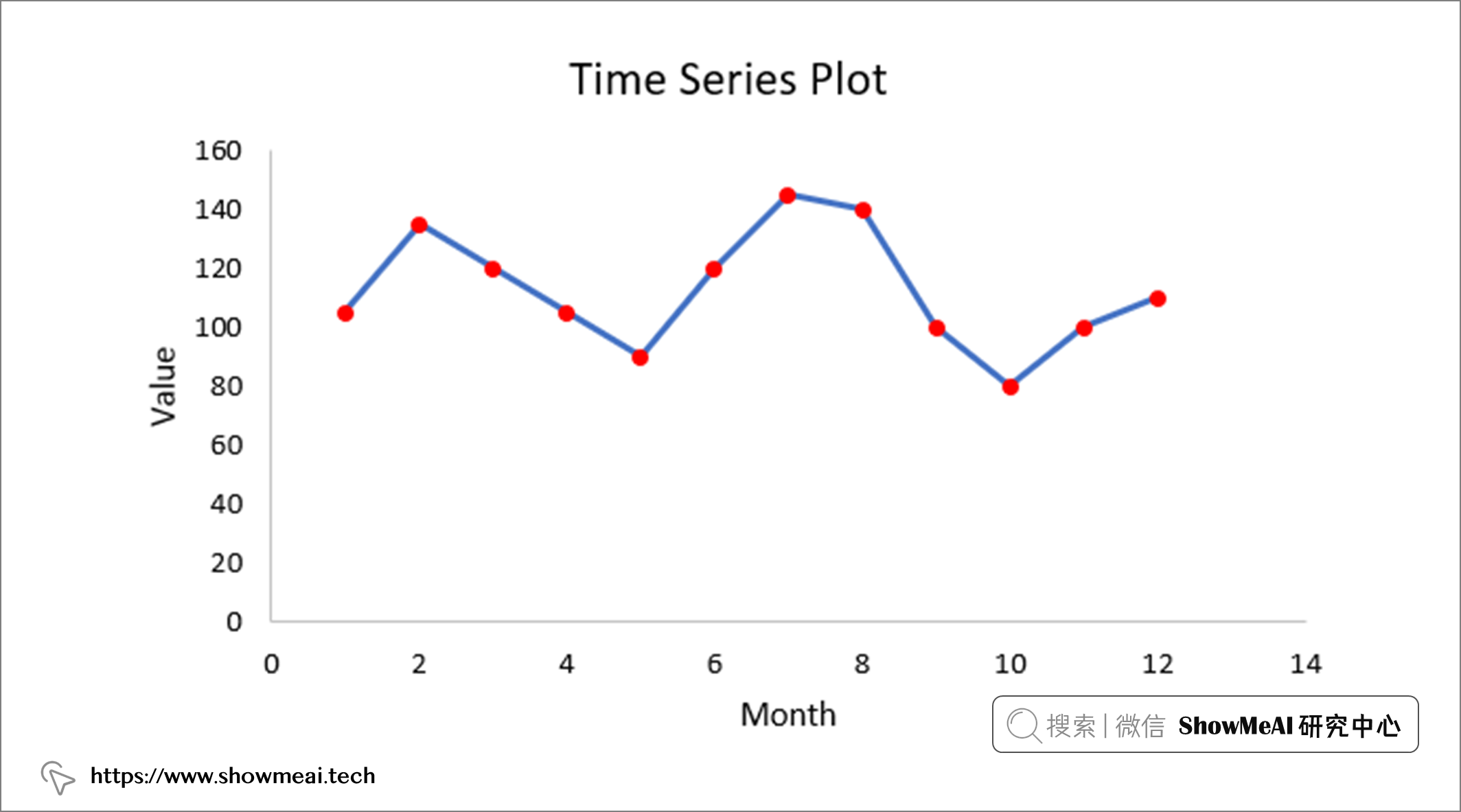```python

# 構建時長為7的時間序列

pd.date_range("2022-01-01", periods=7, freq='D')

```

```python

# 設置隨機種子，可以復現

np.random.seed(12)

# 構建數據集

df = pd.DataFrame({ 'date': pd.date_range("2022-01-01", periods=180, freq='D'), 'sales': np.random.randint(1000, 10000, size=180)})

# 設置索引 df = df.set_index('date') ```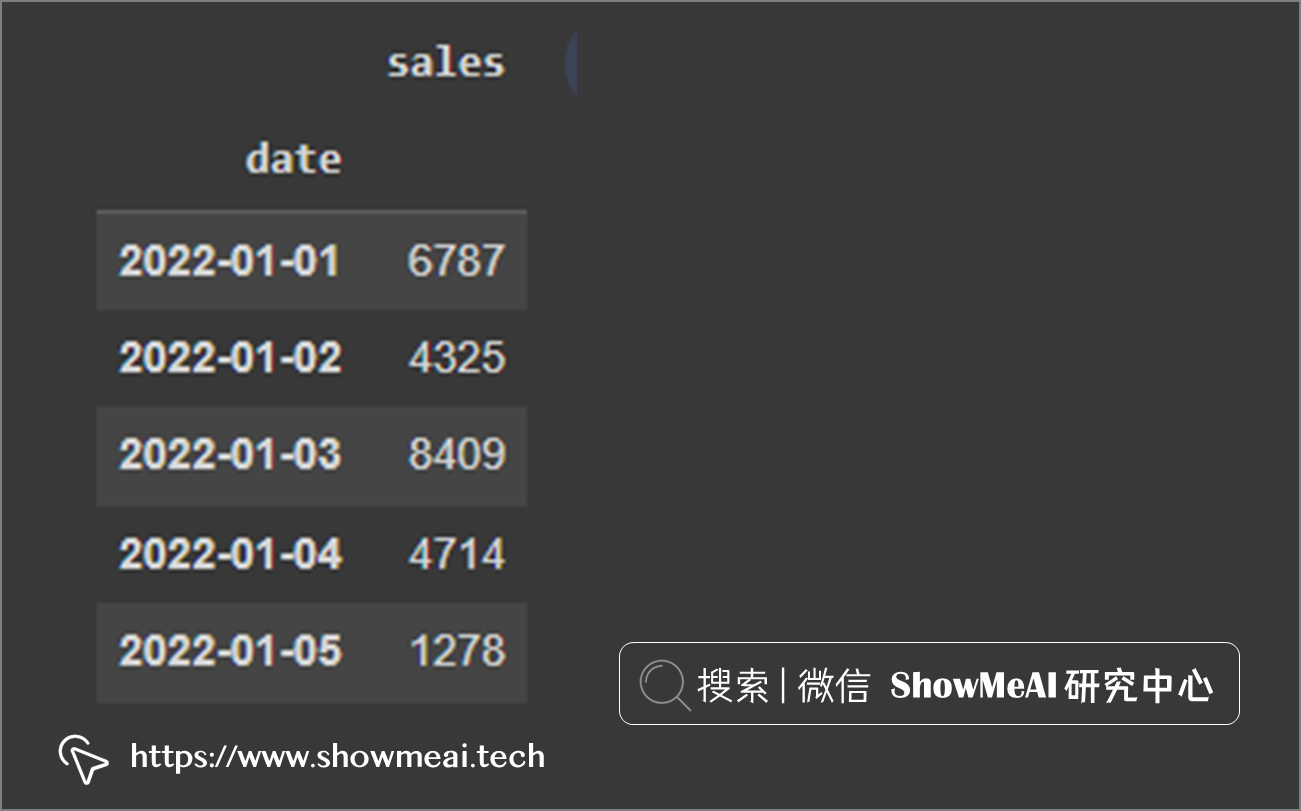## 💦 重採樣

Pandas 中很重要的一個核心功能是`resample`，重新採樣，是對原樣本重新處理的一個方法，是一個對常規時間序列數據重新採樣和頻率轉換的便捷的方法。

```python DataFrame.resample(rule, how=None, axis=0, fill_method=None, closed=None, label=None, convention='start',kind=None, loffset=None, limit=None, base=0)```

```python

# Resample by month end date

df.resample(rule= 'M').mean() ```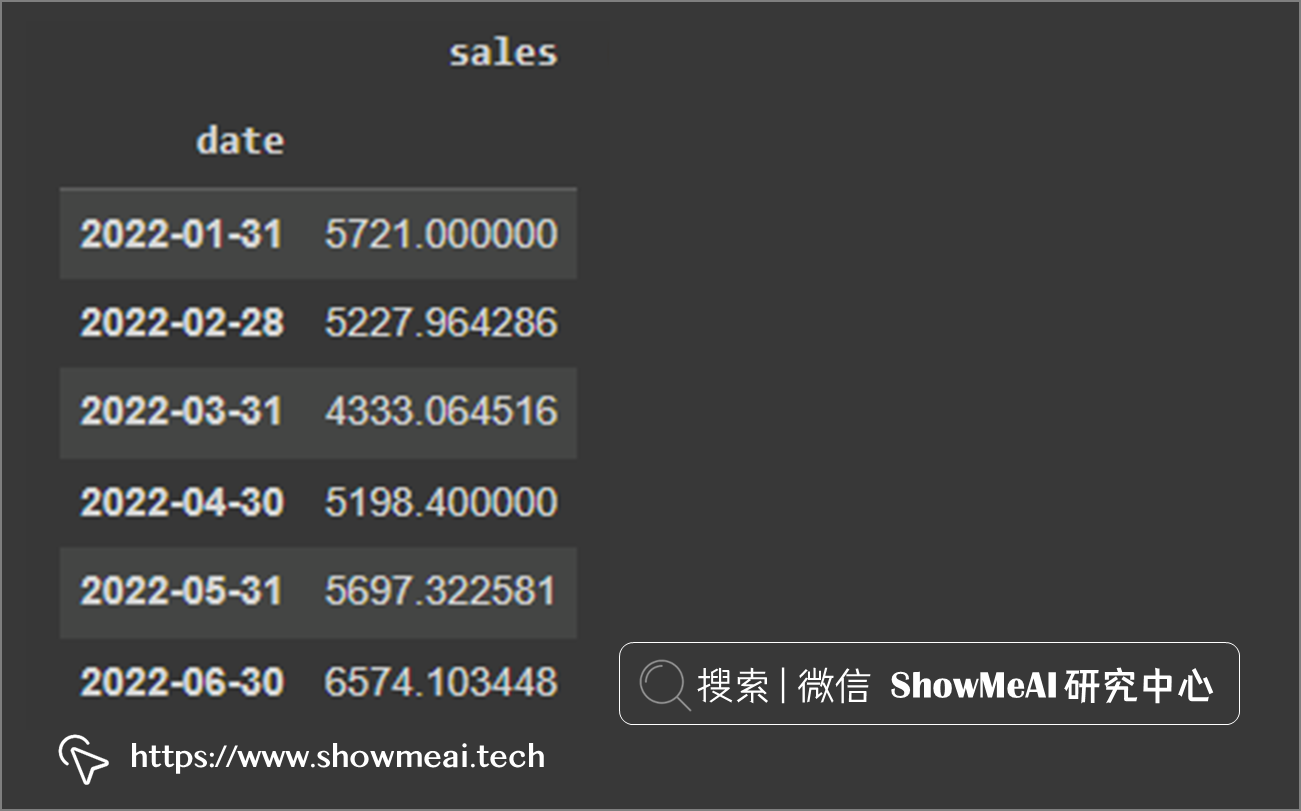```python

# 採樣繪圖

df.resample('W').mean().plot(figsize=(15,5), title='Avg Weekly Sales'); ```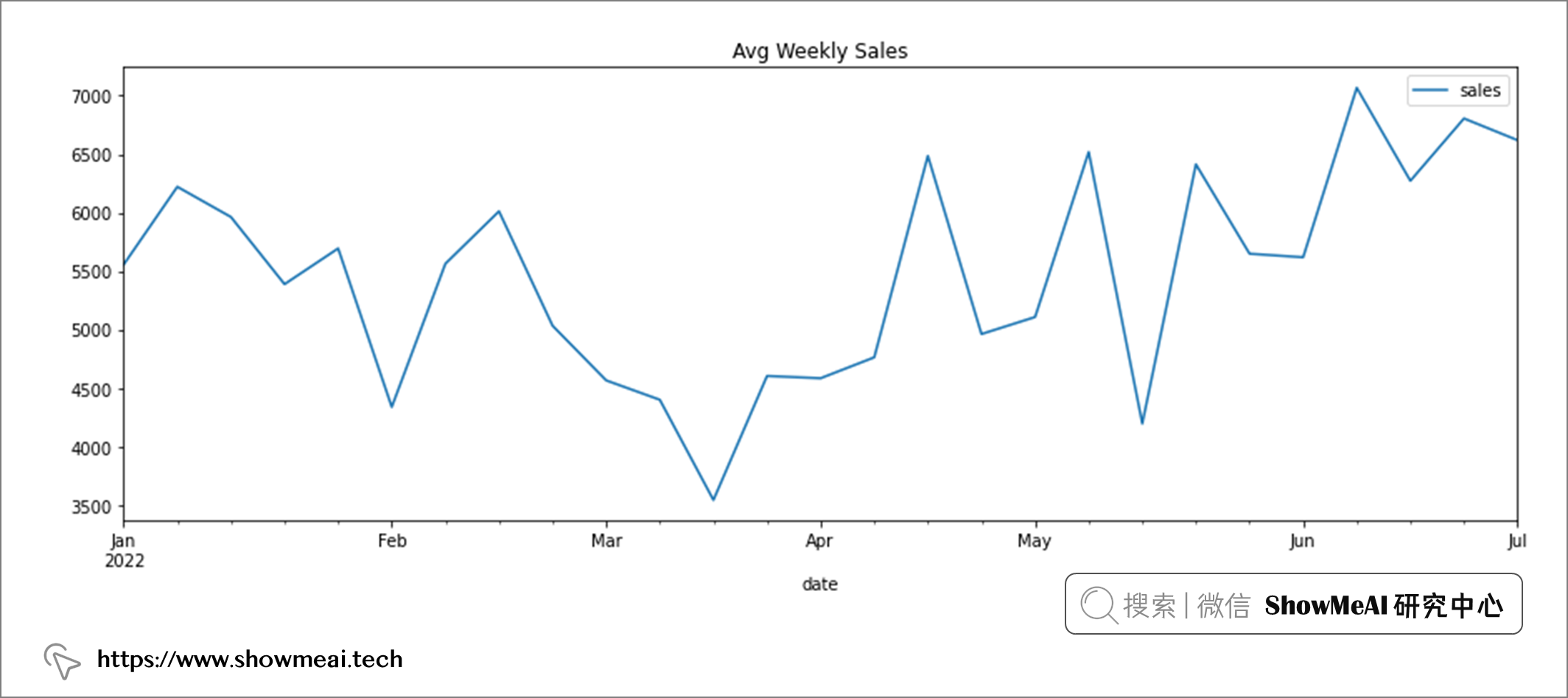## 💦 平移

Pandas 中的`shift`功能，可以讓字段向上或向下平移數據。這個平移數據的功能很容易幫助我們得到前一天或者後一天的數據，可以通過設置`shift`的參數來完成上週或者下週數據的平移。

```python

# 原始數據的一份拷貝

df_shift = df.copy()

# 平移一天

df_shift['next_day_sales'] = df_shift.sales.shift(-1)

# 平移一週

df_shift['next_week_sales'] = df_shift.sales.shift(-7) ```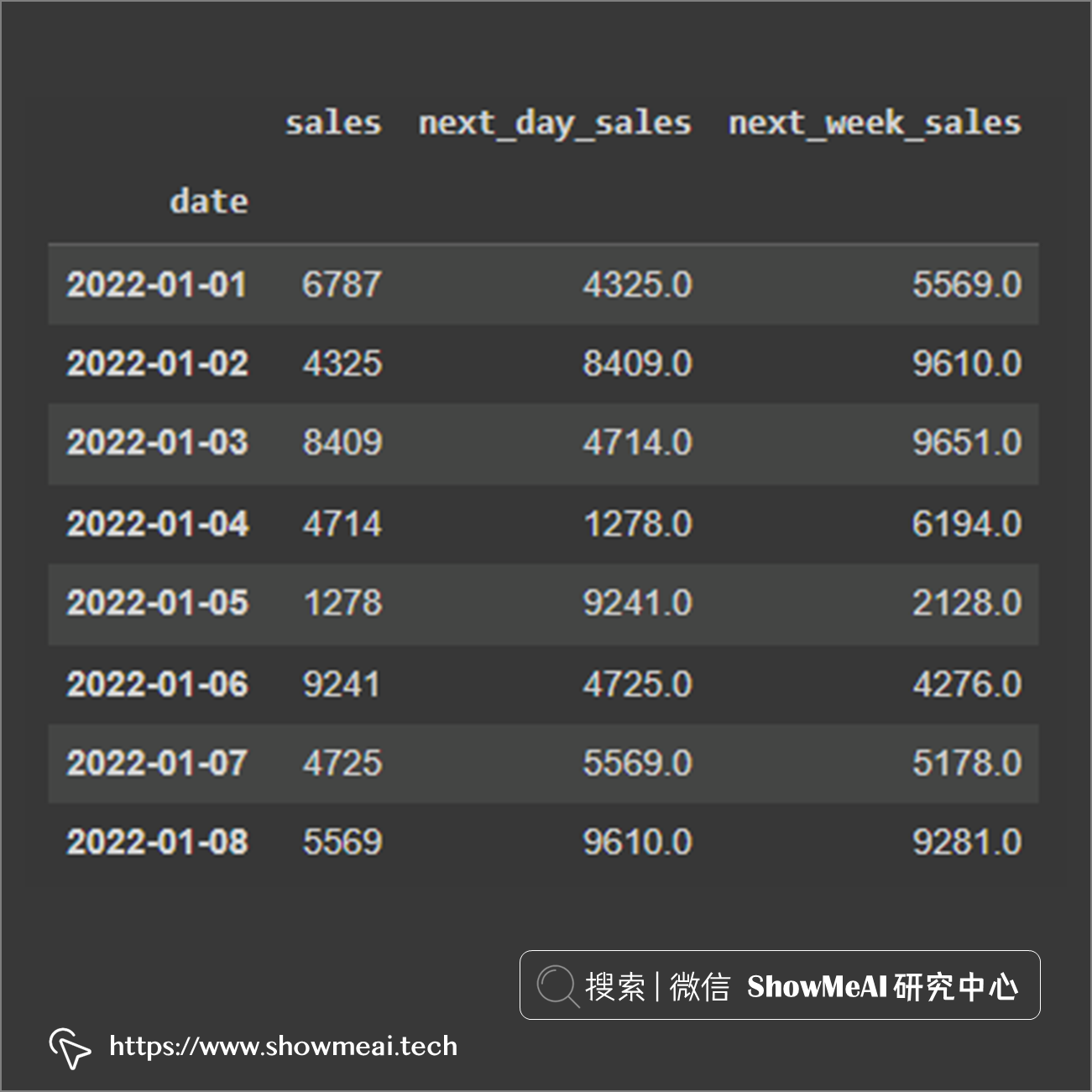```python

# 計算差值

df_shift['one_week_net'] = df_shift.sales - df_shift.sales.shift(-7) ```

```python

# 長度為2天的窗口，求滑動平均

df.rolling(2).mean() ```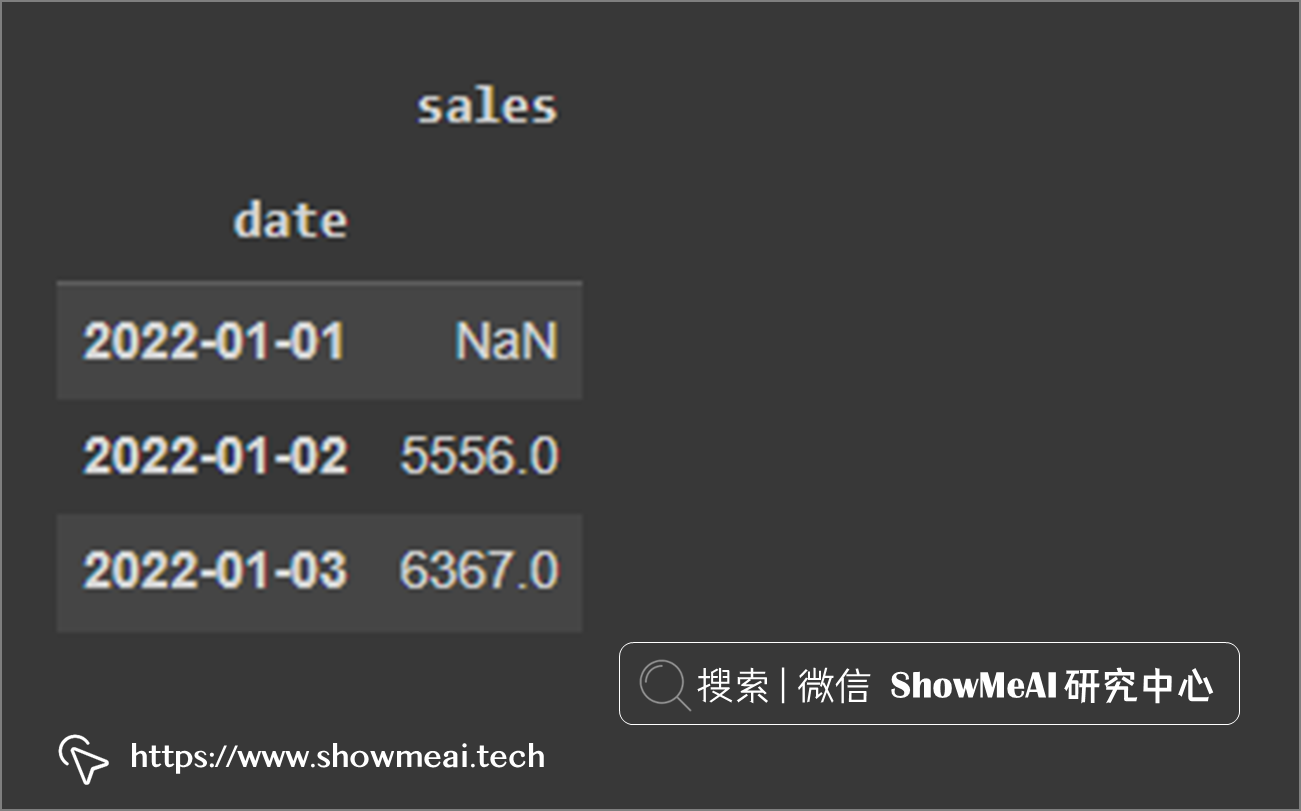```python

# 滑動平均繪圖

df.sales.plot(figsize=(25,8), legend=True, linestyle='--', color='darkgray') df.rolling(window=7).sales.mean().plot(legend=True, label='7 day average', linewidth=2) df.rolling(30).sales.mean().plot(legend=True, label='30 day average', linewidth=3) df.rolling(100).sales.mean().plot(legend=True, label='100 day average', linewidth=4) ```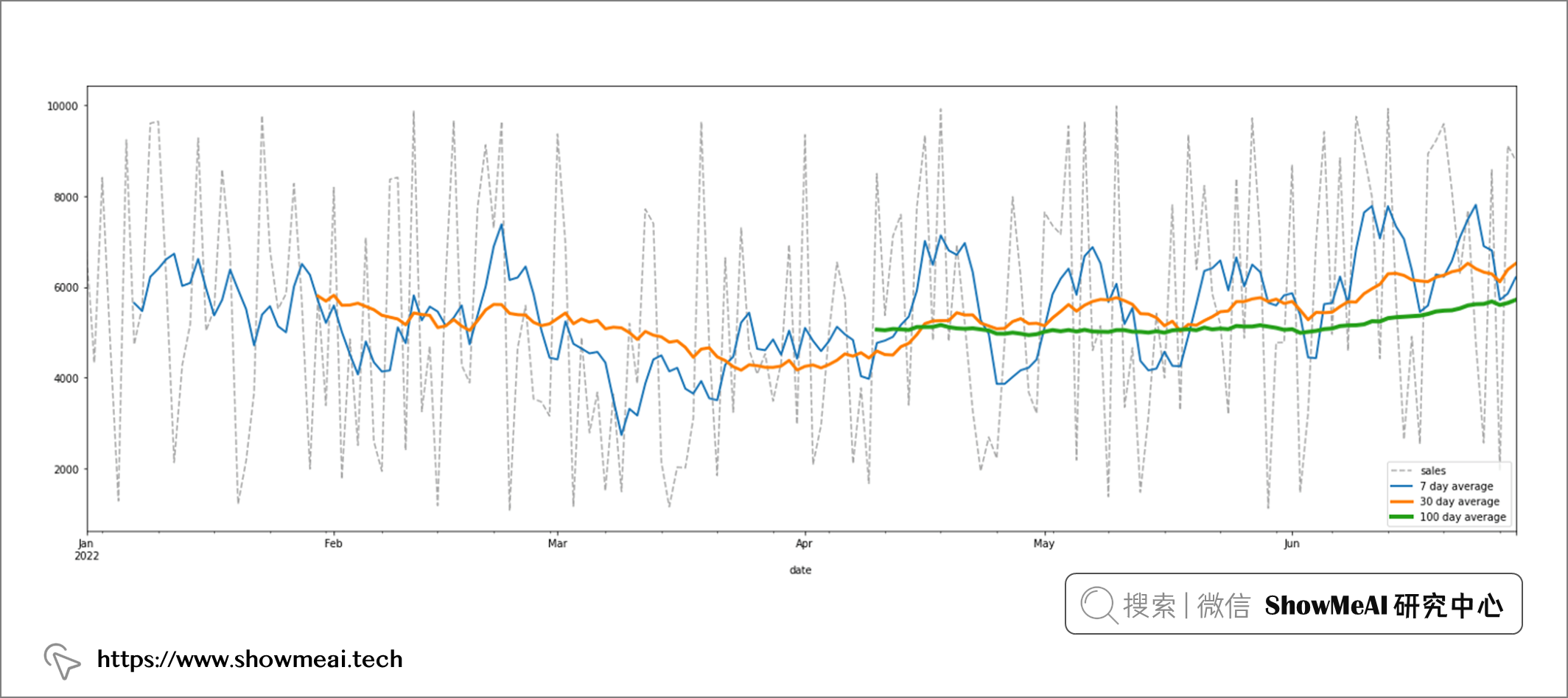# 💡 總結

Pandas在時間序列處理和分析中也非常有效，ShowMeAI在本篇內容中介紹的3個核心函數，是最常用的時間序列分析功能：

• `resample`：將數據從每日頻率轉換為其他時間頻率。
• `shift`：字段上下平移數據以進行比較或計算。
• `rolling`：創建滑動平均值，查看趨勢。

# 參考資料